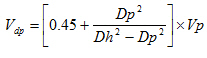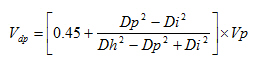# Surge and Swab Calculation Method 1

This is the first method to determine surge and swab pressure.

Surge is additional pressure due to pipe movement downward and swab is reduction of pressure due to upward movement of drill string.Bottom hole pressure is reduced due to swabbing effect.Bottom hole pressure is increased due to surging effect.

The calculation steps are as follows:

1. Determine pressure around drill pipe

2. Determine pressure loss around drill collar

3. Determine total pressure loss by summation of the figures in the 1st and 2nd step

4. Determine surge and swab pressure

The First Step: determine pressure loss around drill pipe

1. Determine nWhere;

n is the power law exponent.

Θ600 is a value at 600 viscometer dial reading.

Θ300 is a value at 300 viscometer dial reading.

2. Determine KWhere;

K is the fluid consistency unit

Θ300 is a value at 300 viscometer dial reading.

n is the power law exponent.

3. Determine fluid velocity around drill pipe

For closed-ended pipe (plugged flow)For open-ended pipeWhere;

Vdp is the fluid velocity around drill pipe in ft/min.

Vp is pipe movement velocity in ft/min.

Dp is drill pipe diameter in inch.

Dh is hole diameter in inch.

Di is inner diameter of drill pipe in inch.

4. Maximum pipe velocity

Vm = Vdp x 1.5

Where;

Vdp is the fluid velocity around drill pipe in ft/min.

Vm is maximum pipe velocity.

5. Pressure loss around drill pipeThe Second Step: determine pressure loss around drill collar

You also need to consider pressure loss around drill collar or BHA as well because they have different OD which sometimes creates significant surge/swab pressure.

1. Determine fluid velocity around drill collar

For close-ended pipe (plugged flow)For open-ended pipeWhere;

Vdc is the fluid velocity around drill collar in ft/min.

Dc is drill collar diameter in inch.

Dh is hole diameter in inch.

Dci is inner diameter of drill collar in inch.

2. Maximum pipe velocity

Vm = Vdc x 1.5

Where;

Vdc is the fluid velocity around drill collar in ft/min.

Vm is maximum pipe velocity.

3. Pressure loss around drill collarThe Third Step: Total Pressure Loss = Pdp + Pdc

The Final Step:

For surge pressure, you need to add this figure to hydrostatic pressure of mud.

Bottom hole pressure = Hydrostatic pressure + Total Pressure Loss

On the other hand, if you want to get swab pressure, the pressure sure must be subtracted from the hydrostatic pressure.

Bottom hole pressure = Hydrostatic pressure – Total Pressure Loss

Ref books:

Lapeyrouse, N.J., 2002. Formulas and calculations for drilling, production and workover, Boston: Gulf Professional publishing.

Share the joy
Tagged , , , . Bookmark the permalink.Working in the oil field and loving to share knowledge.

### 4 Responses to Surge and Swab Calculation Method 1

1.Rick Gade says:

I don’t understand why there is a 0.45 added to the ratio of the drill pipe and hole size ratios.

Vdp = [0.45 + Dp^2/(Dh^2 – Dp^2)] x Vp

Why shouldn’t it be:

Vdp = Dp^2/(Dh^2 – Dp^2) x Vp

Thanks,
Rick

•DrillingFormulas.Com says:

Rick,

This is derived from the actual test from the author.

Regards,
Shyne

This site uses Akismet to reduce spam. Learn how your comment data is processed.